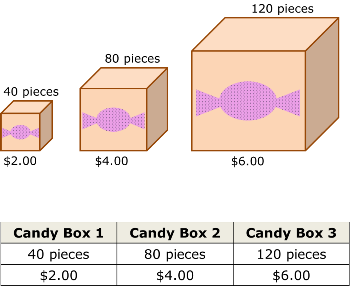# Identifying the Constant of Proportionality

An error occurred trying to load this video.

Try refreshing the page, or contact customer support.

Coming up next: Representing Proportional Relationships by Equations

### You're on a roll. Keep up the good work!

Replay
Your next lesson will play in 10 seconds
• 0:00 Identifying the…
• 1:22 Constant of…
• 2:05 Word Problems
• 3:11 Diagrams and Tables
• 4:17 Graphs
• 5:12 Lesson Summary

Want to watch this again later?

Timeline
Autoplay
Autoplay
Speed

#### Recommended Lessons and Courses for You

Lesson Transcript
Instructor: Damien Howard

Damien has a master's degree in physics and has taught physics lab to college students.

Constants of proportionality might sound like something complicated, but chances are, you've encountered them before. In this lesson, we'll explore the constant of proportionality and how to find it.

## Identifying the Constant of Proportionality

One Saturday morning, you find yourself at the local grocery store helping out with a little shopping for your family. You've been asked to go pick up a can of tomatoes. When you get to the aisle, you see the brand you want on sale: two for \$3.00. In your head, you divide three by two and know the tomato can will cost \$1.50. What you just did, without even knowing it, is find the constant of proportionality for the cost of a tomato can.

The constant of proportionality is the ratio between two directly proportional quantities. In our tomato example, that ratio is \$3.00/2, which equals \$1.50. Two quantities are directly proportional when they increase and decrease at the same rate. When you buy more or less, the total price of the tomato cans in this example goes up or down correspondingly.

Even though the amount of tomato cans bought and the total price can change, the price for a single tomato can, our constant of proportionality, remains the same. This is why we call the constant of proportionality a constant, a number with a fixed value.

In this lesson, we're going to look at multiple ways to identify the constant of proportionality. We'll see how to identify it in equations, word problems, diagrams, tables, and graphs.

## Constant of Proportionality Equation

Earlier we mentioned that the ratio for the constant of proportionality in our example was \$3.00/2. We can use this ratio as an example to find a general equation for any constant of proportionality. The constant of proportionality (k) equals the total price (y) divided by the number of cans (x).

k = y/x

Or, the total price equals the constant of proportionality multiplied by the number of cans:

y = kx

The second formula is a common alternate form of the constant of proportionality formula. While working with the equations, just replace the total price and number of cans with whatever two other directly proportional quantities you have in your problem.

## Word Problems

When trying to find the constant of proportionality, you're not always going to begin with an equation. This is especially true when it comes to speech. In a math class, we replicate verbal descriptions with word problems. Let's look at an example of this.

Chris mows 3 acres of his parent's farmland in 12 hours. Over the next several days, he mows 8 more acres in a total of 32 hours. How many hours does Chris spend per acre mowed?

Here, the constant of proportionality ratio we're trying to find is how many hours it takes to mow an acre. In other words, hours divided by acres mowed. We have two situations to test: 3 acres in 12 hours and 8 acres in 32 hours.

k = y/x

1) k = 12/3 = 4 hours per acre

2) k = 32/8 = 4 hours per acre

We can see it takes Chris 4 hours to mow a single acre. This is our constant because no matter how many acres he mows, or how much time he spends mowing, it will always take 4 hours to mow a single acre.

## Diagrams and Tables

We just used a word problem to find the constant of proportionality, but in other situations, you might be asked to find one through a visual diagram or a table.

Diagrams and tables like the ones below work exactly the same way for finding the constant of proportionality. If we compare the two, we can see that each column of the table is like one image in the diagram.To unlock this lesson you must be a Study.com Member.

### Register to view this lesson

Are you a student or a teacher?

### Unlock Your Education

#### See for yourself why 30 million people use Study.com

##### Become a Study.com member and start learning now.
Back
What teachers are saying about Study.com

### Earning College Credit

Did you know… We have over 160 college courses that prepare you to earn credit by exam that is accepted by over 1,500 colleges and universities. You can test out of the first two years of college and save thousands off your degree. Anyone can earn credit-by-exam regardless of age or education level.